# Correlation matrix

Correlation matrix shows correlation coefficients for column pairs of a given dataset. TableTorch supports both the Pearson and the Spearman’s rank coefficients.

In the following sections, some variants of correlation matrices are computed for the vehicle dataset. The video presented below demonstrates how to use TableTorch to produce a colorful correlation matrix right in a spreadsheet.

Watch on YouTube: Compute a correlation matrix in Google Sheets with TableTorch 4:33

## Start TableTorch

1. Install TableTorch to Google Sheets via Google Workspace Marketplace. More details on initial setup.
2. Click on the TableTorch iconon right-side panel of Google Sheets.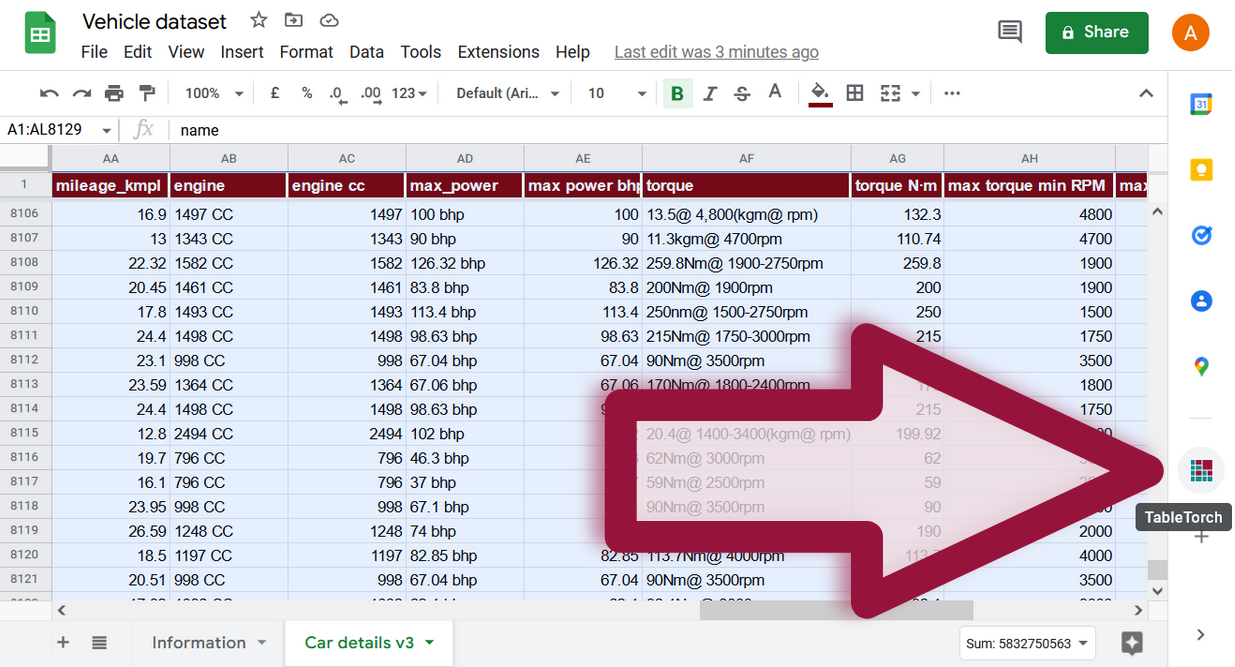## Pearson coefficients for every column pair

Select the whole range of the sheet and click Correlation matrix menu item inside TableTorch.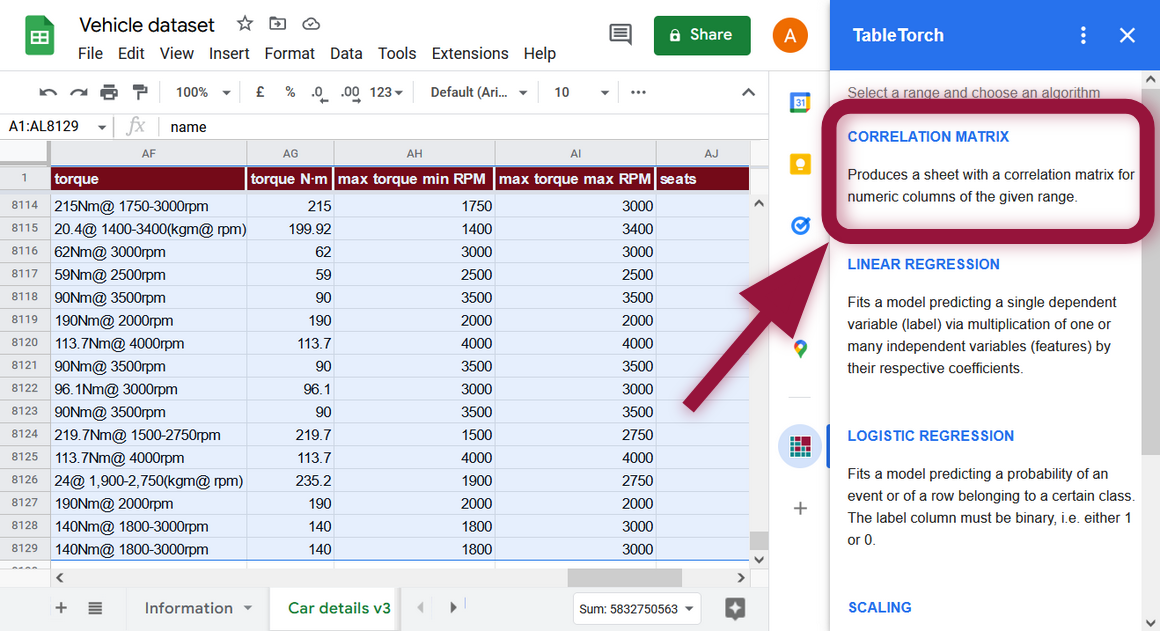Non-numeric columns will be automatically filtered out.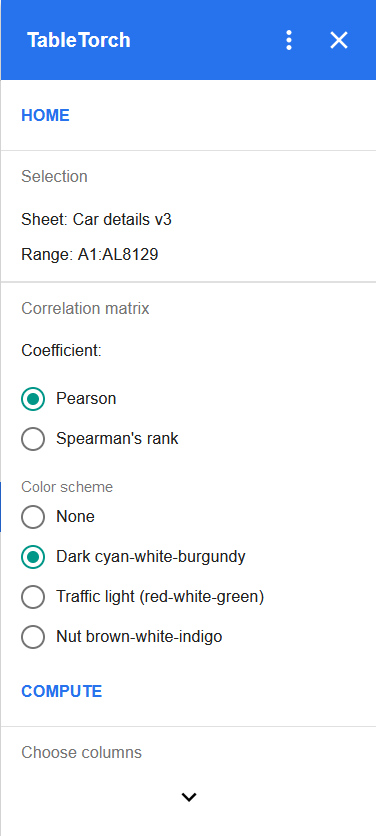Click on the Compute button to produce a new sheet with the correlation matrix. The produced matrix will look like the following one: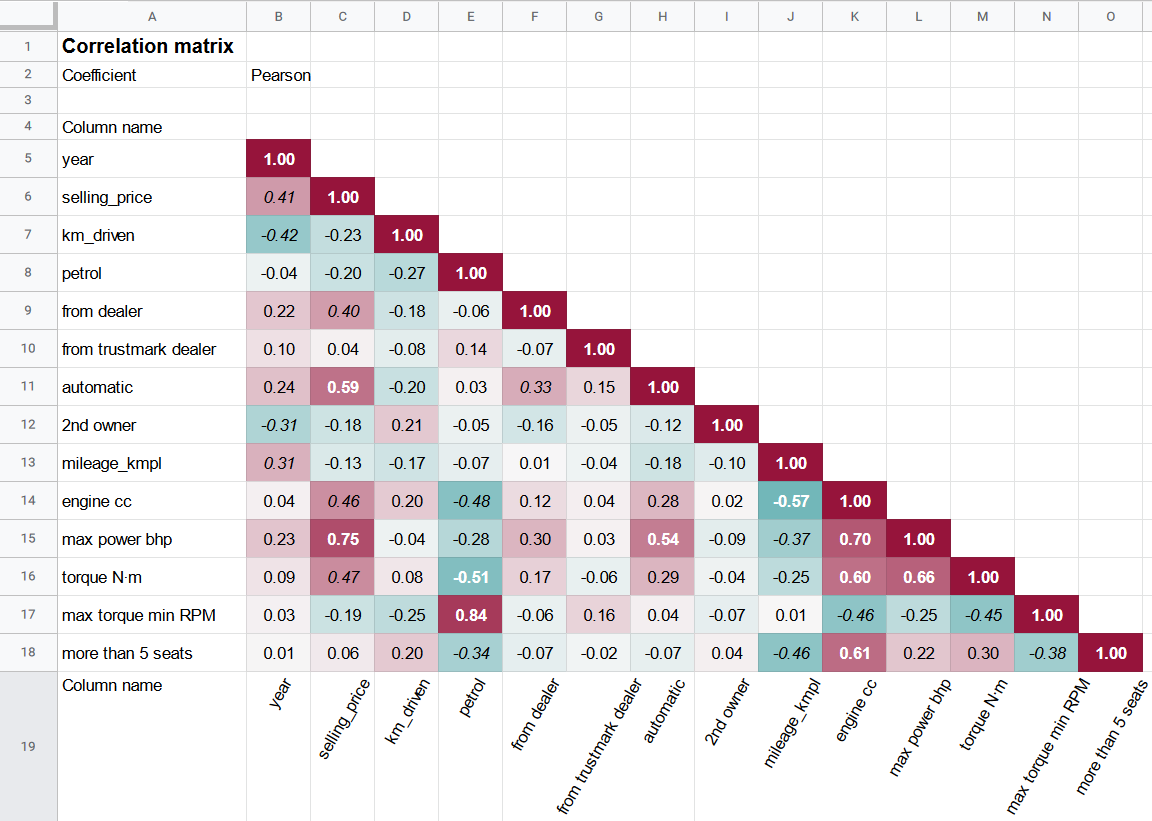The default settings produce an already useful matrix. For example, if the aim is to do a regression in order to be able to predict the value of selling_price column, it is possible to draw the following conclusions from the produced matrix:

1. `selling_price` is negligibly correlated with `from trustmark dealer` column suggesting that it wouldn’t make sense to use this feature in a regression. It is same with the `more than 5 seats` column.
2. `petrol` is highly correlated with `max torque min RPM` indicating that it would make sense to use only one of those features in a regression of `selling_price`.
3. The same could be true for the `engine cc` and `max power bhp` columns.

## Configuration

TableTorch can be configured to produce different matrices via editing the following settings: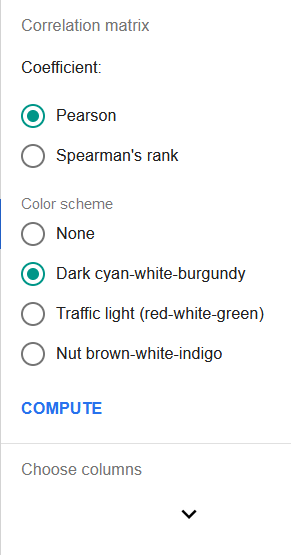• Coefficient: either Pearson or Spearman’s rank. If you would like to rank-scale only certain columns before computing the coefficients, use the standalone Scaling function of the TableTorch before creating a correlation matrix.
• Color scheme: whether and how to highlight the cells of the produced matrix.
• Choose columns: by default, TableTorch will calculate the coefficients for every single column pair of the table. However, it is possible to select only the needed columns using this section.

## Spearman’s rank coefficients for specified column pairs

Simply put, Spearman’s rank coefficient is a Pearson coefficient applied to rank-scaled column data. TableTorch uses fractional ranking which is equivalent to `RANK.AVG(value, data, TRUE)` Google Sheets function, i.e. two identical values will be assigned their average rank. The produced correlation matrix will thus identify non-linear correlations as well as the linear ones. However, it shall be noted that TableTorch’s Linear regression implementation does not support automatic rank-scaling. Therefore, if some strong non-linear correlations are identified, it is advisable to use standalone Scaling and run the regression against scaled data.

TableTorch produces following matrix with Spearman’s rank coefficients for select columns: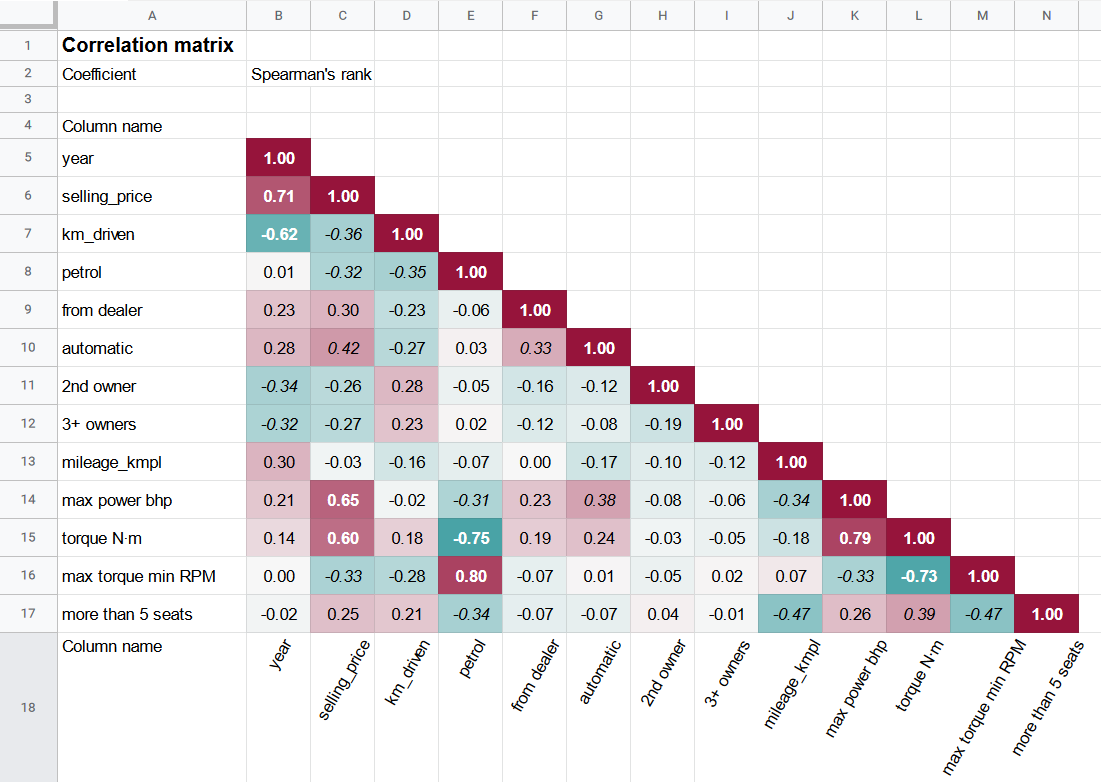## Conclusion

Having reviewed the correlation matrix for the given data, it is now possible to do a linear regression in order to fit a model predicting desired variable.